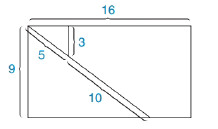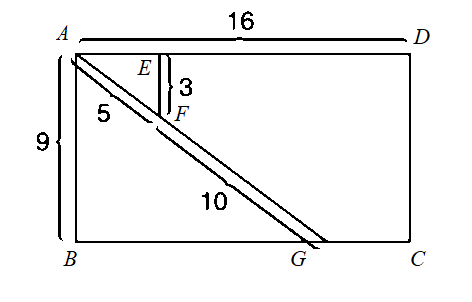Chapter 5.4, Problem 38EElementary Geometry For College St...

7th Edition
Alexander + 2 others
ISBN: 9781337614085

Solutions

Chapter
SectionElementary Geometry For College St...

7th Edition
Alexander + 2 others
ISBN: 9781337614085
Textbook Problem

When the rectangle in the accompanying drawing (whose dimensions are 16 by 9) is cut into pieces and rearranged, a square can be formed. What is the perimeter of this square?To determine

To Find:

The perimeter of square,.

Explanation

Given the rectangle in the accompanying drawing (whose dimensions are 16 by 9) is cut into pieces and rearranged, a square can be formed.

The given figure,

From the figure, ΔAEF and ΔABG are right triangle with right angle E and B.

From ΔAEF is right angle with hypotenuse AF,

AF2=AE2+EF252=AE2+3225=AE2+9AE2=259AE2=16AE=16AE=4

Then the length of side ED is,

Still sussing out bartleby?

Check out a sample textbook solution.

See a sample solution

The Solution to Your Study Problems

Bartleby provides explanations to thousands of textbook problems written by our experts, many with advanced degrees!

Get Started

If the point (3, 7) is on the graph of f, then f(3) = __________.

Precalculus: Mathematics for Calculus (Standalone Book)

Calculate y'. 35. y = cot(3x2 + 5)

Calculus: Early Transcendentals

Find the value of the sum. 25. n=120(1)n

Single Variable Calculus: Early Transcendentals, Volume I

In Exercises 516, evaluate the given quantity. log416

Finite Mathematics and Applied Calculus (MindTap Course List)

limx6(x210x)=. a) 24 b) 6 c) 26 d) does not exist

Study Guide for Stewart's Single Variable Calculus: Early Transcendentals, 8th

True or False: Du f(a, b) = ∇(a, b) · u.

Study Guide for Stewart's Multivariable Calculus, 8th